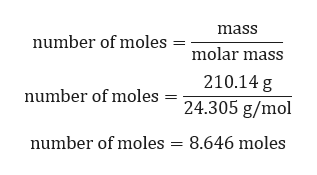# You are running a rather large scale reaction where you prepare the grignard reagent phenylmagnesium bromide by reacting 210.14 grams of magnesium with 772 ml of bromobenzene. How many moles of grignard reagent would you expect to form? (the density of bromobenzene is 1.495 g/ml, Mg = 24.3 g/mol, bromobenzene=157.01 g/mol)

Question

You are running a rather large scale reaction where you prepare the grignard reagent phenylmagnesium bromide by reacting 210.14 grams of magnesium with 772 ml of bromobenzene. How many moles of grignard reagent would you expect to form? (the density of bromobenzene is 1.495 g/ml, Mg = 24.3 g/mol, bromobenzene=157.01 g/mol)

check_circle

Step 1

Given:

Mass of Mg = 210.14 g

Volume of bromobenzene = 772 ml

the density of bromobenzene = 1.495 g/ml

Chemical reaction is given by,

Step 2

Calculation for mass of bromobenzene:

Mass = density × volume

Mass = 1.495 g/ml × 772 ml

Mass = 1154.14 g.

Step 3

Calculation for number ...help_outlineImage Transcriptionclosemass number of moles molar mass 210.14 g number of moles 24.305 g/mol number of moles 8.646 moles fullscreen

### Want to see the full answer?

See Solution

#### Want to see this answer and more?

Solutions are written by subject experts who are available 24/7. Questions are typically answered within 1 hour.*

See Solution
*Response times may vary by subject and question.
Tagged in

### Chemistry